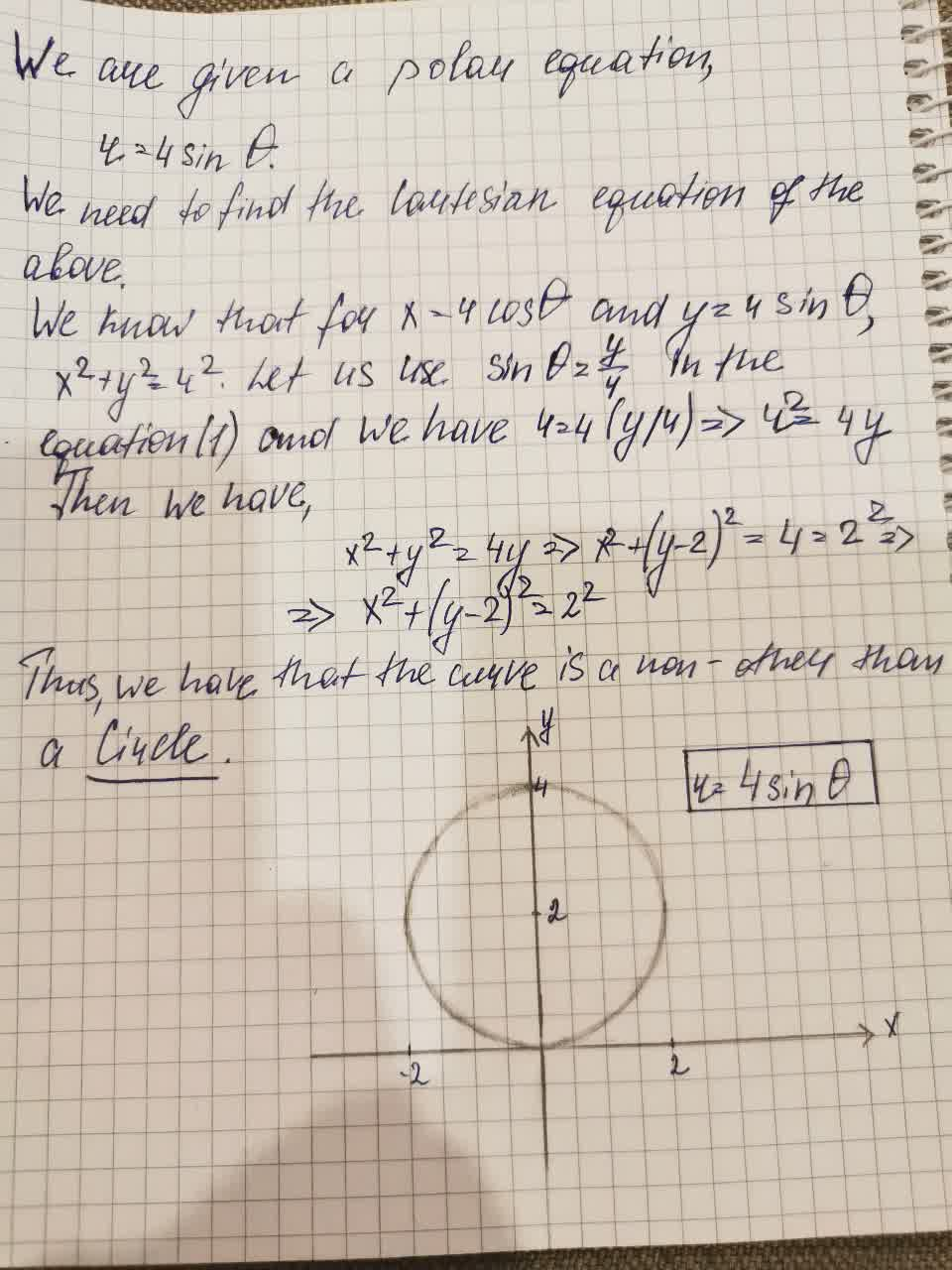Consider the curve in the plane given in polar coordinates by r=4\sin \theta. Find tusagirl007A 2021-08-22 Answered
Consider the curve in the plane given in polar coordinates by $$\displaystyle{r}={4}{\sin{\theta}}$$. Find the cartesian equation for the curve and identify the curve

• Questions are typically answered in as fast as 30 minutes

Solve your problem for the price of one coffee

• Math expert for every subject
• Pay only if we can solve itAamina Herring

Given information: